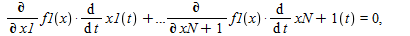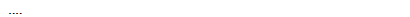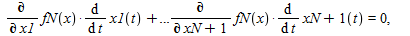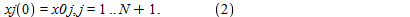:

## The Draghilev method 1. Some mathematical background

Maple

This is an effective method of solving systems of  N nonlinear and nonalgebraic equations in N+1 real-valued variables:
F(x)=0, where F=(f1,f2,..., fN) and x=(x1,x2,...,xN+1). (1)

All the functions fj(x) are assumed to be continuously differentiable in some domain D in R^(N+1).
In general, such systems have an infinite set of the solutions which form a space curve in R^(N+1).
The Optimization and DirectSearch solvers have difficulties with it.
The idea of the Dragilev method consists in the following. Let us assume that the space curve (1) can be parametrized through the  parameter t
which can be taken as the  length of the arc (for example, see http://en.wikipedia.org/wiki/Curve ).
That presentation provides to avoid the difficulties associated with self-intersections and singularities.
We will obtain the coordinates of the points of the space curve under consideration as the solution
of the Cauchy problem for certain system of ODEs. We put the coordinates of a known point of (1) as the initial conditions.
The system of ODEs is obtained by the differentiation  with respect to t:We treat (2) as a linear system in the derivatives diff(xj(t),t),j=1..N+1. In general, its solutions form an infinite set.
We choose the  solution of (2) as follows. We put diff(xN+1)= -Determinant(Matrix(n, (i,j)->diff(fi(x),xj))).
This is one of the key points of the method under consideration.
Next, we find diff(xj,t), j=1..N. At last, we numerically solve that system of ODEs, obtaining an array of points.
The general case of a system of N nonlinear  equations in N variables
G(y)=0, where y=(y1, y2, ..., yN), G=(g1,g2,..., gN) (3),
is reduced to the previous one by homotopy. We choose a point y0= (y01, ..., y0N) and consider the system
G(y)- v*G(y0)=0 (4)
of N equations in N+1 variables (v is taken as  a dummy variable). Changing v from 1 to 0, we arrive to (3).
We don't consider any mathematical justification of the above pattern here as that does not deal with Maple.
I would like to point out the sites

http://forum.exponenta.ru/viewtopic.php?t=3892 and
http://forum.exponenta.ru/viewtopic.php?t=11284 , where the Dragilev method is discussed. Unfortunately, in Russian.
The googling of "Dragilev" and "Draghilev" does not produce many info.
A short biographical sketch: Dr. Anatoliy Vladimirovich Dragilev (1923 - 1997) was a professor at  Rostov State University in Russia.
See his articles here: http://www.mathnet.ru/php/person.phtml?&personid=32359&option_lang=eng and
http://www.zentralblatt-math.org/zmath/en/search/?q=au%3A%22dragilev%2C%20a*%20v*%22 .

I am very grateful to Professor Alexey B. Ivanov, who is an enthusiast of the Dragilev method, for the very useful discussions.﻿